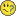New to QlikView

Discussion board where members can get started with QlikView.

HighlightedNot applicable

Subtotals pivot table

Hello, I am fairly new to Qlikview.

In my pivot table I want to show the sales for Brands. But I only want to show it in Cities with a total sales above a certain amount. After some tries I managed to get this situation in my pivot table (see table "Sales if total" in the attached file). Unfortunatly the subtotals are wrong in this table. The subtotals are calculated including the values of the excluded items. Can someone please help me with the solution to this problem?

Tags (3)
1 Solution

Accepted SolutionsMVP

Re: Subtotals pivot table

Hi RazRep72

You can try the below expression

sum(aggr(if(sum(TOTAL <Country, City> Sales)>= \$(vSum),

sum(Sales)), Country,City, Brand))

Find the attachment.

5 RepliesMVP

Re: Subtotals pivot table

Hi RazRep72

You can try the below expression

sum(aggr(if(sum(TOTAL <Country, City> Sales)>= \$(vSum),

sum(Sales)), Country,City, Brand))

Find the attachment.Not applicable

Re: Subtotals pivot table

Thanks settu_periasamy‌! That works great.

As I'm new to Qlikview, i'm taking things step by step, trying to understand what is happening. As you probably saw there is another variable for date. If I try putting the variable date in your expression, the pivot table has the same problem as before. The pivot table shows the correct data, but the subtotals are wrong.

This is the expression I used:

if(

sum(TOTAL <Country, City> {<Date={"<=\$(vDate)"}>} Sales)>= \$(vSum),

sum(aggr( sum({<Date={"<=\$(vDate)"}>} Sales), Country, City, Brand))

)

I thought I understood how the expression works, but it seems I don't.MVP

Re: Subtotals pivot table

Hi,

You can try this expression

=sum(aggr(if(sum({<Date={"<=\$(vDate)"}>}TOTAL <Country, City> Sales)>= \$(vSum),

sum({<Date={"<=\$(vDate)"}>}Sales)), Country,City, Brand))find the attachment.Not applicable

Re: Subtotals pivot table

Thanks again settu_periasamy‌!

Seems I still have to learn a lot about the order of the expressions used. Is there a good explanation about this somewhere out there?MVP

Re: Subtotals pivot table

Hi RazRep72

Some times we don't get the expected result. We need to try with different way. May be you can check with Community search like 'Set analysis with if'. There is lot of documents available.

I'm learning things thru community.